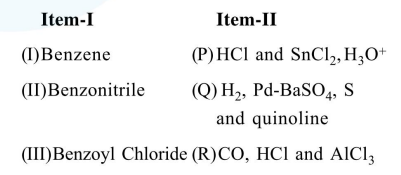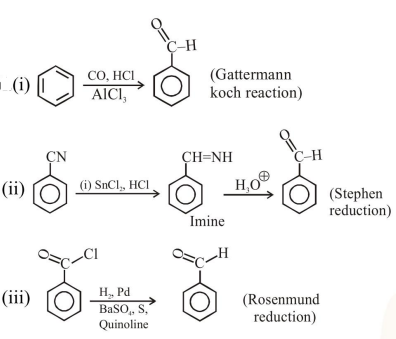# The correct match between Item-I (starting material)

Question:

The correct match between Item-I (starting material) and Item-II (reagent) for the preparation of benzaldehyde is :1. (I)-(Q), (II)-(R) and (III)-(P)

2. (I)-(R), (II)-(Q) and (III)-(P)

3. (I)-(R), (II)-(P) and (III)-(Q)

4. (I)-(P), (II)-(Q) and (III)-(R)

Correct Option: , 3

Solution: